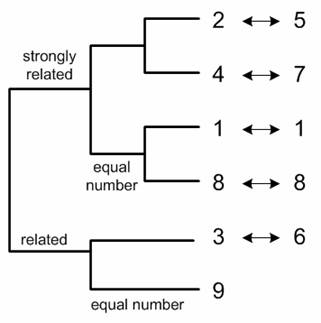## Pattern of Division

Comparing division table and the multiplication table , we can see that some sequence in rows of division table is exactly the same sequence in rows of multiplication table. For example, row sequence of 4 in division table is equal to row sequence of 7 in multiplication table. The same things happen in row sequence of 7 in division table is equal to row sequence of 4 in multiplication table. In this case we say that digital root 4 is related to digital root 7. Through this comparison we can cluster digital roots into several groups. Digital root 2 and 5 is strongly related because the sequence in division table and multiplication table are equal. Digital root 1 and digital root 8 are strongly related to itself because the sequence in division table and multiplication table are equal. Digital root 3 and 6 does not produce the same sequence between division table and multiplication table, but they are related because the division table contains the number of its matched. Similar situation happen in digital root 9. It does not produce the same sequence between division table and multiplication table, but they are related because the division table contains the number of its matched. However, digital root 9 is only related to itself, not to other number.in the next section, we will discuss about more exciting pattern of the power of digital root.

Preferable reference for this tutorial is

Teknomo, Kardi (2005). Digital Root. https:\\people.revoledu.com\kardi\tutorial\DigitSum\